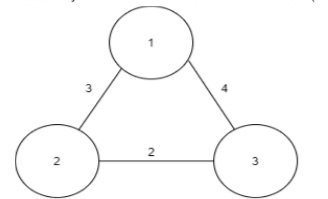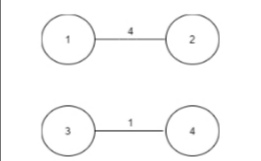3

# Minimum Travel Time

Difficulty: MEDIUM
Avg. time to solve
25 min
Success Rate
75%

Problem Statement
Suggest Edit

#### If there is no possible way to travel all the roads, then return -1.

##### Input Format:
``````The first line of the input contains an integer, 'T,’ denoting the number of test cases.
The first line of each test case contains two space-separated integers, 'N' and 'M', denoting the number of cities and the number of bidirectional roads respectively.
The next 'M' lines of each test case contain the description of the 'M' roads.
The 'i'th' line contains three space-separated integers 'City1', 'City2', and 'Time'. 'City1' and 'City2' denote the two cities that are connected by the 'i'th' road, and 'time' denotes the time it takes to travel between the two cities using this road.
``````
##### Output Format:
``````The only line of output of each test case should contain the minimum time that Mr. X will take to visit all the roads satisfying the above conditions. If there is no way to visit all the roads, then print -1.
Print the output of each test case in a new line.
``````
##### Note :
``````You do not need to print anything. It has already been taken care of. Just implement the given function.
``````
##### Constraints:
``````1 <= T <= 10
1 <= N <= 10
0 <= M  <= (N*(N-1))/2
1 <= City1, City2 <= N
1 <= Time <= 10^6

Any two cities are directly connected by at most one road.

Where 'T' denotes the number of test cases, 'N' denotes the number of cities, 'M' denotes the number of roads, 'City1' and 'City2' denotes the two cities that are connected by the 'i'th' road, and 'Time' denotes the time it takes to travel between the two cities.

Time Limit: 1 sec
``````
##### Sample Input 1:
``````2
3 3
1 2 3
1 3 4
2 3 2
4 2
1 2 4
3 4 1
``````
##### Sample Output 1:
``````9
-1
``````
##### Explanation for Sample Input 1:
``````For the first test case :
Ninja Land has the below structure. Mr. X can start from the 1st city and can visit all the roads in cyclic order. The total travel time will be 9 ( 4 + 3 + 2) in this case
````````````For the second test case:
It can be seen that it is impossible to travel through all the roads using the given roads. Hence, the answer is -1 in this case.
``````##### Sample Input 2:
``````2
4 4
1 2 5
3 1 3
2 3 6
3 4 5
4 6
3 4 1
3 2 5
1 2 6
1 3 4
2 4 3
1 4 2
``````
##### Sample Output 2:
``````24
27
``````Console